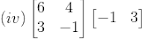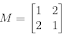# ICSE Solutions for Selina Concise Chapter 9 Matrices Class 10 Maths

### Exercise 9(A)

1. State, whether the following statements are true or false. If false, give a reason.

(i) If A and B are two matrices of orders 3×2 and 2×3 respectively; then their sum A + B is possible.

(ii) The matrices A2×3 and B2×3 are conformable for subtraction.

(iii) Transpose of a 2×1 matrix is a 2×1 matrix.

(iv) Transpose of a square matrix is a square matrix.

(v) A column matrix has many columns and one row.

Solution

(i) False.

The sum of matrices A + B is possible only when the order of both the matrices A and B are same.

(ii) True

(iii) False. Transpose of a 2 ×1 matrix is a 1×2 matrix.

(iv) True

(v) False. A column matrix has only one column and many rows.

Solution

If two matrices are said to be equal, then their corresponding elements are also equal.

∴ x = 3,

y + 2 = 1 so, y = -1

z – 1 = 2 so, z = 3

3. Solve for a, b and c if

Solution

If two matrices are said to be equal, then their corresponding elements are also equal.

Then,

(i) a + 5 = 2 ⇒ a = -3

-4 = b + 4 ⇒ b = -8

2 = c – 1 ⇒ c = 3

(ii) a = 3

a – b = -1

⇒ b = a + 1 = 4

b + c = 2

⇒ c = 2 – b = 2 – 4 = -2

4. If A = [8 -3] and B = [4 -5]; find:

(i) A + B (ii) B – A

Solution

(i) A + B = [8 -3] + [4 -5] = [8+4 -3-5] = [12 -8]

(ii) B – A = [4 -5] – [8 -3] = [4-8 -5-(-3)] = [-4 -2]

(i) B + C

(ii) A – C

(iii) A + B – C

(iv) A – B +C

Solution

### Exercise 9(B)

1. Evaluate:

(i) 3[5 -2]

Solution

3[5 -2] = [3×5 3x-2] = [15 -6]

Solution

Solution

Solution

2. Find x and y if:

(i) 3[4 x] + 2[y -3] = [10 0]

Solution

Taking the L.H.S, we have

3[4 x] + 2[y -3] = [12 3x] + [2y -6] = [(12+2y) (3x–6)]

Now, equating with R.H.S we get

[(12+2y) (3x–6)] = [10 0]

⇒ 12 + 2y = 10 and 3x – 6 = 0

⇒ 2y = -2 and 3x = 6

⇒ y = -1 and x = 2

Solution

We have,

So, equating the matrices we get

-x + 8 = 7 and 2x – 4y = -8

⇒ x = 1 and 2(1) – 4y = -8

⇒ 2 – 4y = -8

⇒ 4y = 10

⇒ y = 5/2

(i) 2A – 3B + C (ii) A + 2C – B

Solution

(i) 2A – 3B + C

(ii) A + 2C – B

Solution

Given,

(i) find the matrix 2A + B.

(ii) find a matrix C such that:

Solution

(i) 2A + B

(ii)

### Exercise 9(C)

1. Evaluate: if possible:

If not possible, give reason.

Solution

= [6 + 0] = 

= [-2+2 3-8] = [0 -5]The multiplication of these matrices is not possible as the rule for the number of columns in the first is not equal to the number of rows in the second matrix.

(i) AB

(ii) BA

(iii) AI

(iv) IB

(v) A2

(vi) B2A

Solution

Solution

A2 =

A2 = B

On comparing corresponding elements, we have

4x = 16

⇒ x = 4

And,

1 = -y

y = -1

4. Find x and y, if:

Solution

On comparing the corresponding terms, we have

5x – 2 = 8

⇒ 5x = 10

⇒ x = 2

And,

20 + 3x = y

⇒  20 + 3(2) = y

20 + 6 = y

y = 26

On comparing the corresponding terms, we have

x = 2

And,

-3 + y = -2

⇒ y = 3 – 2 = 1

(i) (AB) C (ii) A (BC)

Solution

(AB)C

(ii) BC

A(BC)

∴ its seen that (AB)C = A(BC)

(i) AB (ii) BA (iii) A2

Solution

(i) AB

(ii) BA

(iii) A2 = A x A, is not possible since the number of columns of matrix A is not equal to its number of rows.

Solution

8. Ifand I is a unit matrix of the same order as that of M; show that:

M2 = 2M + 3I

Solution

Thus, M2 = 2M + 3I

Solution

So, BA =M2

On comparing the corresponding elements, we have

-2b = -2

⇒ b = 1

And, a = 2

(i) A – B

(ii) A2

(iii) AB

(iv) A2 – AB + 2B

Solution

(iv) A2 – AB + 2B

(i) (A + B)2

(ii) A2 + B2

(iii) Is (A + B)2 = A2 + B2 ?

Solution

So, (A + B)2 = (A + B)(A + B)

A2 + B2

Thus, its seen that (A + B)2 ≠ A2 + B2+

Solution

B2 = B + ½A

½A = B2 – B

Solution

And, given A= I

So on comparing the corresponding terms, we have

1 + a = 1

Thus, a = 0

And, -1 + b = 0

Thus, b = 1

(i) A(B + C) = AB + AC

(ii) (B – A)C = BC – AC.

Solution

(i) A(B + C)

AB + AC

Thus, A(B + C) = AB + AC

(ii) (B – A)C

BC – AC

Thus, (B – A)C = BC – AC

Solution

A2 + BC

### Exercise 9(D)

1. Find x and y, if:

Solution

On comparing the corresponding terms, we have

6x – 10 = 8 and -2x + 14 = 4y

⇒ 6x = 18 and y = (14 – 2x)/4

⇒ x = 3 and y = (14 – 2(3))/4

⇒ y = (14 – 6)/ 4

⇒ y = 8/4 = 2

Thus, x = 3 and y = 2

2. Find x and y, if:

Solution

On comparing the corresponding terms, we have

3x + 18 = 15 and 12x + 77 = 10y

⇒ 3x = -3 and y = (12x + 77)/10

⇒ x = -1 and y = (12(-1) + 77)/10

⇒ y = 65/10 = 6.5

Thus, x = -1 and y = 6.5

(i) x, y ∈ W (whole numbers)

(ii) x, y ∈ Z (integers)

Solution

From the question, we have

x2 + y2 = 25 and -2x2 + y2 = -2

(i) x, y ∈ W (whole numbers)

It can be observed that the above two equations are satisfied when x = 3 and y = 4.

(ii) x, y ∈ Z (integers)

It can be observed that the above two equations are satisfied when x = ± 3 and y = ± 4.

(i) The order of the matrix X.

(ii) The matrix X.

Solution

(i) Let the order of the matrix be a×b.

Then, we know that

Thus, for multiplication of matrices to be possible

a = 2

And, form noticing the order of the resultant matrix

b = 1

On comparing the corresponding terms, we have

2x + y = 7 and

⇒ -3x + 4y = 6

Solving the above two equations, we have

x = 2 and y = 3

5. Evaluate:

Solution

Solution

Given,

3A×M = 2B

And let the order of the matric of M be (a×b)

Now, it’s clearly seen that

a = 2 and b = 1

So, the order of the matrix M is (2 x 1)

Now, on comparing with corresponding elements we have

-3y = -10 and 12x – 9y = 12

⇒ y = 10/3 and 12x – 9(10/3) = 12

⇒ 12x – 30 = 12

⇒ 12x = 42

⇒ x = 42/12 = 7/2

find the values of a, b and c.

Solution

On comparing the corresponding elements, we have

a + 1 = 5 ⇒ a = 4

b + 2 = 0 ⇒ b = -2

-1 – c = 3 ⇒ c = -4

(i) A(BA)

(ii) (AB)B.

Solution

(i) A(BA)

(ii) (AB)B

9. Find x and y, if:

Solution

Thus, on comparing the corresponding terms, we have

2x + 3x = 5 and 2y + 4y = 12

⇒ 5x = 5 and 6y = 12

⇒ x = 1 and y = 2

Solution

Now,

On comparing with the corresponding terms, we have

-28 – 3x = 10

⇒ 3x = -38

⇒ x = -38/3

And, 20 – 3y = -8

⇒ 3y = 28

⇒ y = 28/3

A + X = 2B + C

Solution

12. Find the value of x, given that A2 = B,

Solution

Thus, on comparing the terms we get x = 36.